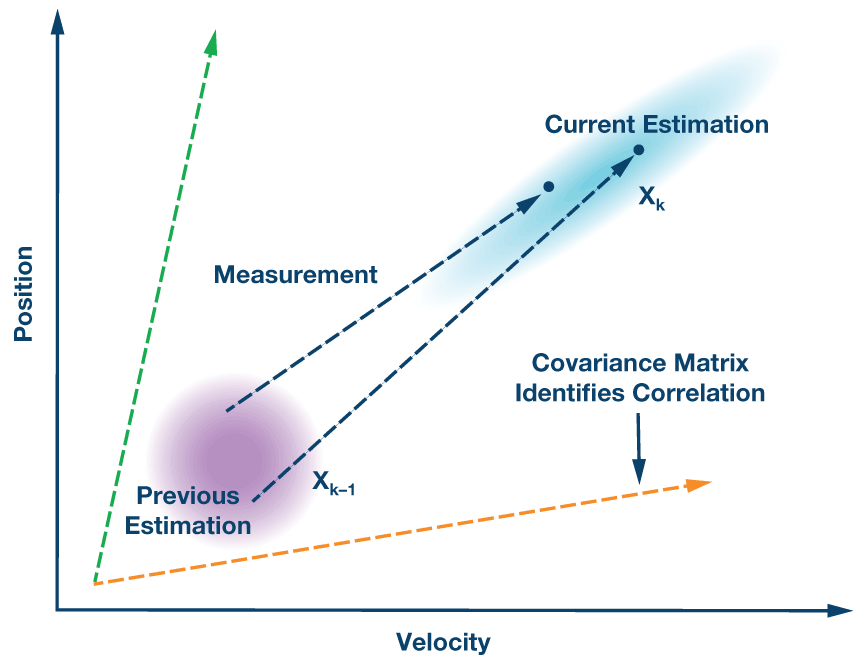# Kalman or FIR Filter for My IMU?

### QUESTION:

In configuring my inertial measurement unit (IMU) for post-filtering of the data after the sensor, I see options for both a decimation FIR filter and also a Kalman filter. Which one is best for my application?

Each of these filter options provides a decidedly different function within the IMU. For the most part, they are independent in their operation. Their use will depend upon the requirements of the end system. Let's take a further look to understand the details and how they can apply to a sensor system.

The use of decimation in conjunction with a finite impulse response (FIR) filter is a method to reduce the full input bandwidth of the IMU in order to focus only on a narrow low-pass frequency band of activity. This can be especially useful when a system is subjected to many rotational and acceleration frequency movements, only a subset of which are important to observe within the sensor. Additionally, any unnecessary or ignored higher frequency activity has the potential to alias back into the band of interest without the filtering rejection of an FIR band-pass filter.

The FIR filter is most valuable when the full bandwidth of the sensor is not needed. Instead, if a known signal frequency bandwidth within a low-pass region is of interest, unwanted signals can be filtered. For example, a system may have a rotational frequency of interest only between 20 Hz to 50 Hz. Although there may be other higher frequency noise that can be detected, it is not of concern for measuring within the IMU. Figure 1 shows a method to use a decimation and FIR filter option B to low-pass filter the full bandwidth by a factor of 16.Figure 1. The FIR filter response of the ADIS16480 (left) and an example use case of the B filter (right) where unwanted signals can be filtered to focus within the low-pass frequency bandwidth of interest.

The Kalman filter, named after electrical engineer coinventor Rudolf Kálmán, provides a different benefit to that of the decimation and FIR filter combination. The word "filter" describing the Kalman filter may actually be a bit of a misnomer. It is more akin to a "recursive estimator." The Kalman filter is most valuable in systems where a predicted location can be more useful than an otherwise unfiltered noisy solution that could have positional error. The Kalman filter estimates orientation angles using all of the sensor axis contributions within the IMU.

Although much more complex than a single equation, we can simplify the use case here by dropping out the state matrices and we can obtain the math shown below:

Xk = Kk × Zk + (1 – Kk) × Xk–1

Xk = Current estimation

Kk = Kalman gain

Zk = Measured value

Xk–1 = Previous estimation

We can treat each k as identifying discrete time intervals or samples of each sensor axis output. The new best estimation is a prediction made from the previous best estimation, plus a gain correction weighting for known external influences. The initial Kalman gain or covariance coefficients are used within the IMU register settings to establish expected correlation between the Isensor output vectors. The optimal covariance values to use within the IMU can often depend on the specific observations. Therefore, it can be an iterative process of measuring, observing data, analysis, adjustment, and repeating. The ADIS16480 incorporates an internal algorithm, using innovation residuals, which can adaptively adjust the covariance terms in real time.Figure 2. An example Kalman filter model where two variables—in this case, velocity and position—have some amount of correlation. A current position can be estimated based upon the previous position, a measured acceleration value from the IMU, and the covariance weighting of the correlation between the two.